## Solving Simple Linear Equations

Algebraic equations are translated from complete English sentences. These equations can be solved. In fact, in order to successfully solve a word problem, an equation must be written and solved.

Look at these two definitions in the following sections and compare the examples to ensure you know the distinction between an expression and an equation.

## Defining an Algebraic Expression

An algebraic expression is a collection of constants, variables, symbols of operations, and grouping symbols, as shown in Example 1.

Example 1: 4( x − 3) + 6

## Defining an Algebraic Equation

An algebraic equation is a statement that two algebraic expressions are equal, as shown in Example 2.

Example 2: 4( x − 3) + 6 = 14 + 2 x

The easiest way to distinguish a math problem as an equation is to notice an equals sign.

In Example 3, you take the algebraic expression given in Example 1 and simplify it to review the process of simplification. An algebraic expression is simplified by using the distributive property and combining like terms.

Example 3: Simplify the following expression: 4( x − 3) + 6

Here is how you simplify this expression:

1. Remove the parentheses using the distributive property.

4 x + −12 + 6

2. Combine like terms.

The simplified expression is 4 x + −6.

Note: This problem does not solve for x. This is because the original problem is an expression, not an equation, and, therefore, cannot be solved.

In order to solve an equation, follow these steps:

1. Simplify both sides of the equation by using the distributive property and combining like terms, if possible.

2. Move all terms with variables to one side of the equation using the addition property of equations, and then simplify.

3. Move the constants to the other side of the equation using the addition property of equations and simplify.

4. Divide by the coefficient using the multiplication property of equations.

In Example 4, you solve the equation given in Example 2, using the four preceding steps to find the solution to the equation.

Example 4: Solve the following equation: 4( x − 3) + 6 = 14 + 2 x

Use the four steps to solving a linear equation, as follows:

• 1.

Distribute and combine like terms.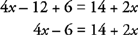• 2a.

Move all terms with variables to the left side of the equation.

In this example, add a −2x to each side of the equation.The addition property of equations states that if the same term is added to both sides of the equation, the equation remains a true statement. The addition property of equations also holds true for subtracting the same term from both sides of the equation.

• 2b.

Place like terms adjacent to each other and simplify.

Note: Subtracting 6 is changed to adding −6 because the commutative property of addition works only if all operations are addition.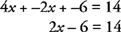• 3.

Move the constants to the right side of the equation and simplify.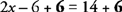Note: The opposite operation was used to move the constant.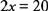• 4.

Divide by the coefficient and simplify.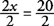The solution is x = 10.

Example 5: Solve the following equation: 12 + 2(3 x − 7) = 5 x − 4

Use the four steps to solving a linear equation, as follows:

• 1a.

Distribute and combine like terms.• 1b.

Place like terms adjacent to each other and simplify.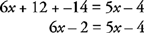• 2a.

Move variables to the left side of the equation.

In this example, add −5 x to each side of the equation.• 2b.

Place like terms adjacent to each other and simplify.

Note: All subtractions are changed to addition of a negative number.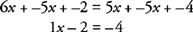• 3.

Move the constants to the right side of the equation and simplify.Note: The opposite operation was used to move the constant.

• 4.

Because the coefficient is 1, Step 4 is not necessary.

The solution is x = −2.

Example 5: Solve the following equation: 6 − 3(2 − x) = −5 x + 40

Use the four steps to solving a linear equation, as follows:

• 1.

Distribute and combine like terms.Did you remember to distribute the negative three?• 2a.

Move variables to the left side of the equation.

In this example, add 5 x to each side of the equation.• 2b.

Place like terms adjacent to each other.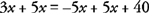• 2c.

Simplify by combining like terms.• 3.

This step is not necessary in this example because all of the constants are on the right side of the equation.

• 4.

Divide by the coefficient and simplify.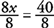The solution is x = 5.

Remember: The four steps for solving equations must be done in order, but not all steps are necessary in every problem.